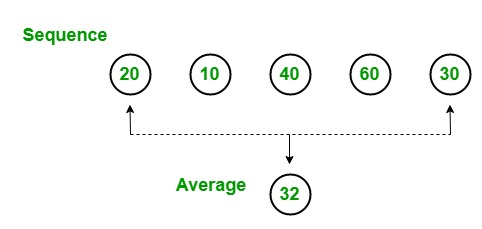# LINQ | How to find average of the given sequence?

In LINQ, you can find the average of the given sequence by using the Average method. This method returns the average of the elements present in the given sequence or collection. It returns nullable, non-nullable decimal, double, floats, int, etc. values. It does not support query syntax in C#, but it supports in VB.NET. It is available in both Enumerable and Queryable classes in C#.Example 1:

 `// C# program to find the average  ` `// the given array ` `using` `System; ` `using` `System.Linq; ` ` `  `class` `GFG { ` ` `  `    ``static` `public` `void` `Main() ` `    ``{ ` ` `  `        ``// Data source ` `        ``int``[] sequence = { 20, 45, 50, 79, 90, 79, 89, 100, 567, 29 }; ` ` `  `        ``// Display the sequence ` `        ``Console.WriteLine(``"The sequence is:"``); ` `        ``foreach``(``int` `s ``in` `sequence) ` `        ``{ ` `            ``Console.WriteLine(s); ` `        ``} ` ` `  `        ``// Finding the average of the element  ` `        ``// present in the given array ` `        ``// Using Average function ` `        ``double` `result = sequence.Average(); ` `        ``Console.WriteLine(``"Average is: {0}"``, result); ` `    ``} ` `} `

Output:

```The sequence is:
20
45
50
79
90
79
89
100
567
29
Average is: 114.8
```

Example 2:

 `// C# program to find the average ` `// salary of the employee ` `using` `System; ` `using` `System.Linq; ` `using` `System.Collections.Generic; ` ` `  `// Employee details ` `class` `Employee { ` ` `  `    ``public` `int` `emp_id ` `    ``{ ` `        ``get``; ` `        ``set``; ` `    ``} ` `    ``public` `string` `emp_name ` `    ``{ ` `        ``get``; ` `        ``set``; ` `    ``} ` `    ``public` `string` `emp_gender ` `    ``{ ` `        ``get``; ` `        ``set``; ` `    ``} ` `    ``public` `string` `emp_hire_date ` `    ``{ ` `        ``get``; ` `        ``set``; ` `    ``} ` `    ``public` `int` `emp_salary ` `    ``{ ` `        ``get``; ` `        ``set``; ` `    ``} ` `} ` ` `  `public` `class` `GFG { ` ` `  `    ``// Main method ` `    ``static` `public` `void` `Main() ` `    ``{ ` `        ``List emp = ``new` `List() { ` `            ``new` `Employee() { emp_id = 209, emp_name = ``"Anjita"``, emp_gender = ``"Female"``, emp_hire_date = ``"12/3/2017"``, emp_salary = 20000 }, ` `                ``new` `Employee() { emp_id = 210, emp_name = ``"Soniya"``, emp_gender = ``"Female"``, emp_hire_date = ``"22/4/2018"``, emp_salary = 30000 }, ` `                ``new` `Employee() { emp_id = 211, emp_name = ``"Rohit"``, emp_gender = ``"Male"``, emp_hire_date = ``"3/5/2016"``, emp_salary = 40000 }, ` `                ``new` `Employee() { emp_id = 212, emp_name = ``"Supriya"``, emp_gender = ``"Female"``, emp_hire_date = ``"4/8/2017"``, emp_salary = 40000 }, ` `                ``new` `Employee() { emp_id = 213, emp_name = ``"Anil"``, emp_gender = ``"Male"``, emp_hire_date = ``"12/1/2016"``, emp_salary = 40000 }, ` `                ``new` `Employee() { emp_id = 214, emp_name = ``"Anju"``, emp_gender = ``"Female"``, emp_hire_date = ``"17/6/2015"``, emp_salary = 50000 }, ` `        ``}; ` ` `  `        ``// Find the average salary of the employee ` `        ``// Using Average () method ` `        ``var` `res = emp.Average(a => a.emp_salary); ` `        ``Console.WriteLine(``"Average salary of the Employees: {0}"``, res); ` `    ``} ` `} `

Output:

```Average salary of the Employees: 36666.6666666667
```

My Personal Notes arrow_drop_upCheck out this Author's contributed articles.

If you like GeeksforGeeks and would like to contribute, you can also write an article using contribute.geeksforgeeks.org or mail your article to contribute@geeksforgeeks.org. See your article appearing on the GeeksforGeeks main page and help other Geeks.

Please Improve this article if you find anything incorrect by clicking on the "Improve Article" button below.

Article Tags :

Be the First to upvote.

Please write to us at contribute@geeksforgeeks.org to report any issue with the above content.